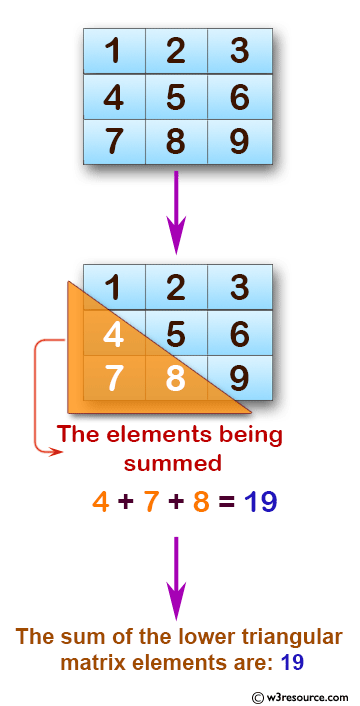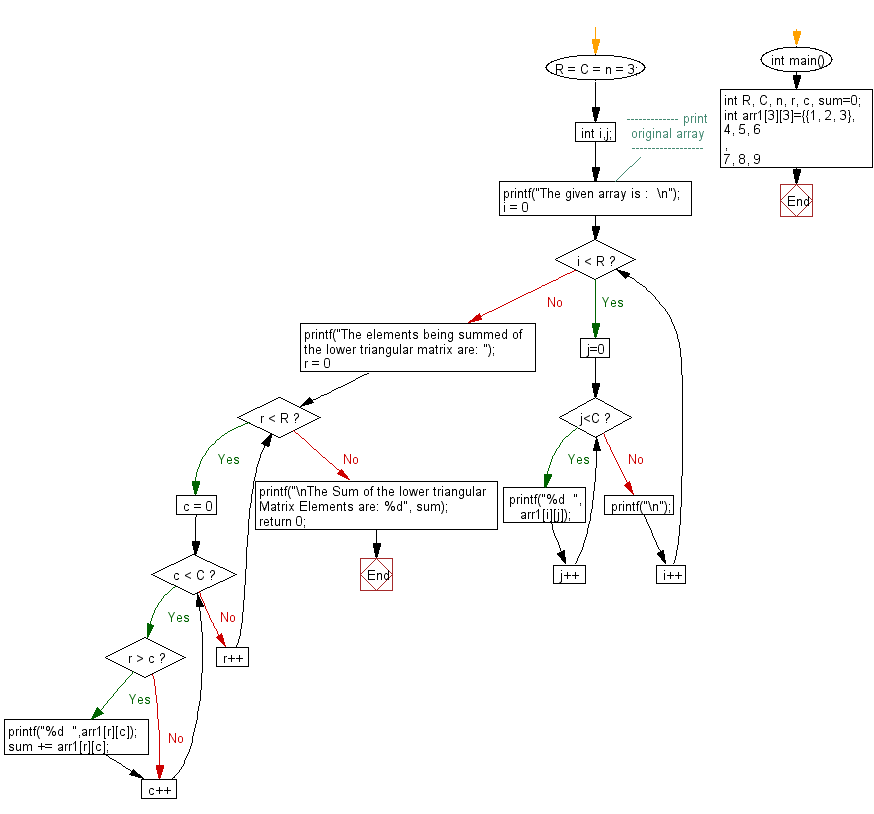﻿ C exercises: Find the sum of lower triangular elements of a matrix - w3resource# C Exercises: Find the sum of lower triangular elements of a matrix

## C Array: Exercise-75 with Solution

Write a program in C to find the sum of lower triangular elements of a matrix.

Pictorial Presentation:Sample Solution:

C Code:

``````#include <stdio.h>
int main()
{
int R, C, n, r, c, sum=0;
int arr1={{1, 2, 3},
{4, 5, 6},
{7, 8, 9}};
R = C = n = 3;
int i,j;
//------------- print original array ------------------
printf("The given array is :  \n");
for(i = 0; i < R; i++)
{
for (j=0;j<C;j++)
{
printf("%d  ", arr1[i][j]);
}
printf("\n");
}
//------------------------------------------------------
printf("The elements being summed of the lower triangular matrix are: ");
for(r = 0; r < R; r++)
{
for(c = 0; c < C; c++)
{
if(r > c)
{
printf("%d  ",arr1[r][c]);
sum += arr1[r][c];
}
}
}
printf("\nThe Sum of the lower triangular Matrix Elements are: %d", sum);
return 0;
}
```
```

Sample Output:

```The given array is :
1  2  3
4  5  6
7  8  9
The elements being summed of the lower triangular matrix are: 4  7  8
The Sum of the lower triangular Matrix Elements are: 19
```

Flowchart:C Programming Code Editor:

Improve this sample solution and post your code through Disqus.

What is the difficulty level of this exercise?

﻿